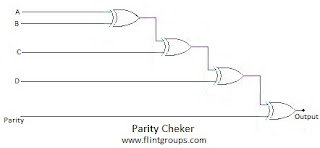# Parity Checker

## Parity Checker

What is Meant by Parity Checker ?
Parity Checker is nothing but used to Check the Parity bits to check the data has been transmitted accurately.The parity bit is added to every data unit that are transmitted using Parity generator. The parity bit for each unit is set so that all bytes have either an odd number or an even number of set bits.

EX-OR Gate is used to Check the parity of a given binary number.If the information contains even number of 1’s the EX-OR gate combination produces the output as ‘0’. Similarly, if the information contains odd number of 1’s, the EX-OR gate combination produces the output as 1.In the Circuit Diagram A 5 bit number with a parity bit drives the input of parity checker. If the binary number applied to it’s input is 01101, the checker produces the output as 1, because the input has odd parity (odd number of ones).

In the digital systems all numbers are either converted into even parity or odd parity before transmission. Hence the parity checker placed at the receiver side can easily find out the errors.

It is the simplest error detection technique. The major disadvantage is that it cannot detect two bit (or even bits) errors, only one bit error (or odd bit error) may be detected.